# Math in Focus Grade 4 Chapter 10 Answer Key Perpendicular and Parallel Line Segments

This handy Math in Focus Grade 4 Workbook Answer Key Chapter 10 Perpendicular and Parallel Line Segments detailed solutions for the textbook questions.

## Math in Focus Grade 4 Chapter 10 Answer Key Perpendicular and Parallel Line Segments

Challenging Practice

In the figure, use a protractor, drawing triangle, and a straightedge to name three pairs of line segments that are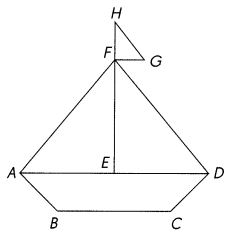Question 1.
perpendicular. ____________________
These three pairs of line segments  HF⊥ FG, FE ⊥ AE, FE ⊥ ED are Perpendicular.

Question 2.
parallel _____________________
These three pairs of line segments FG // AD, AD // BC, HF // FE are Parallel.

Solve.

PQ is a lamp post standing vertically on the ground.
$$\overline{R S}$$ and $$\overline{U T}$$ are horizontal line segments on the ground passing through point Q.
$$\overline{Q T}$$ is perpendicular to $$\overline{Q S}$$.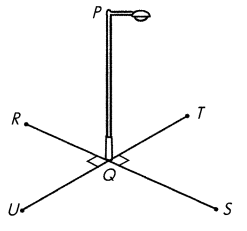Question 3.
Identify two other pairs of line segments that are perpendicular.
The two other pairs of line segments that are perpendicular are RQ ⊥ QU, QU ⊥ QS.

Question 4.
How many right angles are formed at point Q? ____
Four right angles are formed at point Q.

Problem Solving

The diagram shows a road with parallel curbs $$\overline{J K}$$ and $$\overline{L M}$$.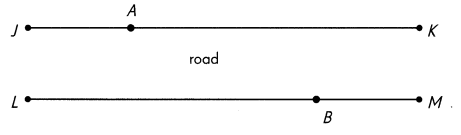Question 1.
Danie is standing at point A and Alicia is standing at point B. They both want to cross the road. Use a drawing triangle to draw the shortest route each can take, and mark all the right angles like this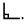Measure the distance along each route.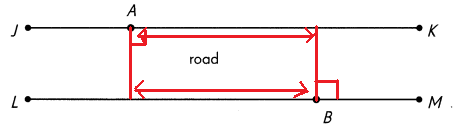Question 2.
What do you know about the distance between parallel line segments?
Parallel line segments are always ____ distance apart.
Parallel line segments are always same distance apart.

Solve.

The cube is placed on a flat surface.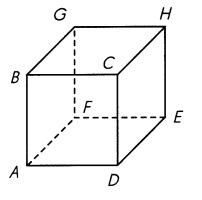Question 3.
How many vertical line segments are there? ____